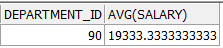# Oracle SQL Tutorials – Chapter 4

Chapter 4 – Group Functions

• AVG Function
• SUM Function
• MIN Function
• MAX Function
• COUNT Function
• DISTINCT Function
• GROUP BY Statement
• HAVING Statement

• One row functions can be applied to a one row of a table and returns a single row.
• But group functions applied to a whole table. Also can be applied to sub-groups, using GROUP BY statement.

AVG & SUM

• Let’s list the average and the sumof the salaries.
```SELECT AVG (salary), SUM (salary)

FROM hr.employees;```MIN & MAX

• Let’s select the minimum and the maximum salary.
```SELECT MIN (salary), MAX (salary)

FROM hr.employees;```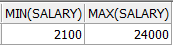COUNT

• Let’s list the count of the employees and how many different positions.
```SELECT COUNT (*), COUNT (DISTINCT job_id)

FROM hr.employees;```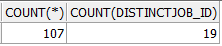DISTINCT

• The Distinct command, groups the data and shows the results just one time.
• Let’s list all the positions.
`SELECT DISTINCT job_id FROM hr.employees;`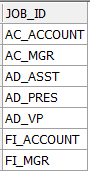GROUP BY STATEMENT

• With this statement, the rows in the table can be divided into smaller groups.
• The columns in the Group By statement, must be added to Select statement.
• The aliases can’t be used in Group By statement.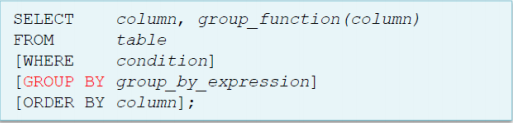• Let’s list the average salaries of departments.
```SELECT department_id, AVG (salary)

FROM hr.employees

GROUP BY department_id

ORDER BY 1;```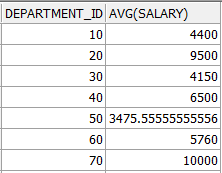• Let’s write another querry using Group By function.
```SELECT department_id,

job_id,

AVG (salary),

SUM (salary),

COUNT (*) Employees

FROM hr.employees

GROUP BY department_id, job_id

ORDER BY department_id;```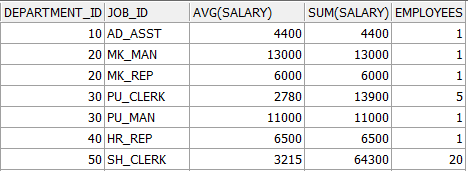HAVING STATEMENT

• Where statement can’t be used with group functions.
• So, the Having statement is used instead of where condition.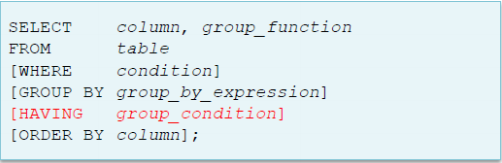• Let’s list the positions which average salaries are higher than 10,000.
```SELECT job_id, AVG (salary)

FROM hr.employees

GROUP BY job_id

HAVING AVG (salary) > 10000;```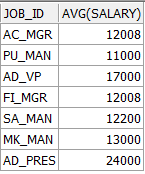• Here is another example of usage having statement.
```SELECT job_id, SUM (salary)

FROM hr.employees

WHERE job_id NOT LIKE '%REP%'

GROUP BY job_id

HAVING SUM (salary) > 13000

ORDER BY SUM (salary);```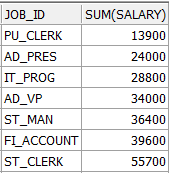NESTED GROUP FUNCTIONS

• Here are two examples and outputs of nested functions.
```SELECT MAX (AVG (salary))

FROM hr.employees

GROUP BY department_id;```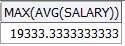```SELECT department_id, AVG (salary)

FROM hr.employees

GROUP BY department_id

HAVING (MAX (salary)) > ALL ( SELECT AVG (salary)

FROM hr.employees

GROUP BY department_id);```